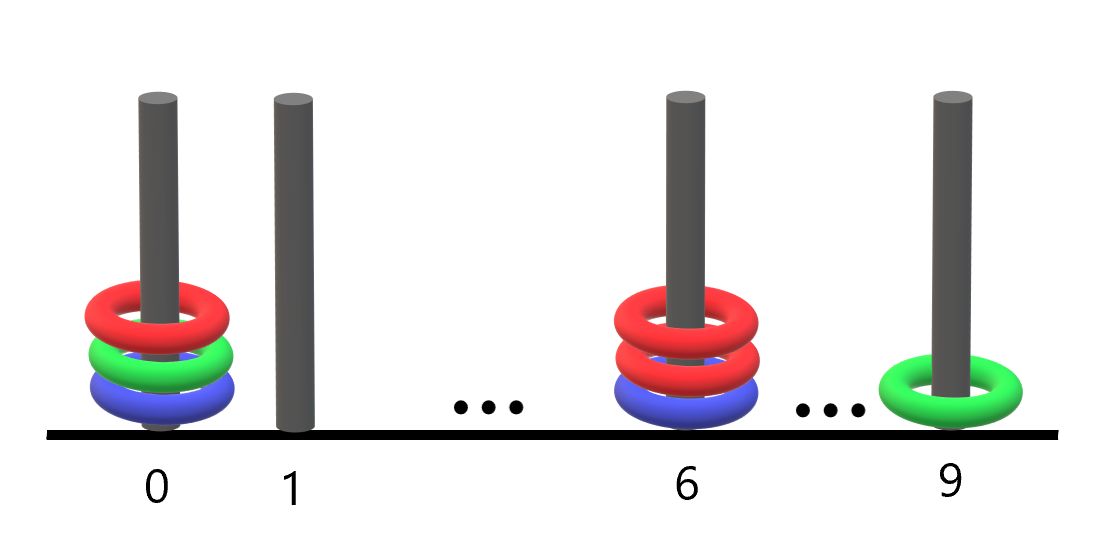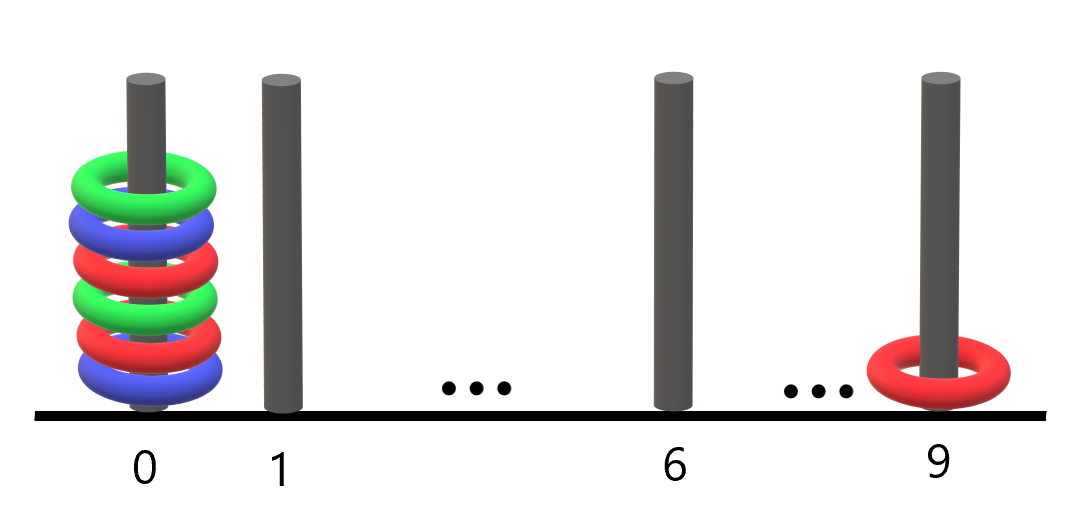2103. Rings and Rods

There are `n` rings and each ring is either red, green, or blue. The rings are distributed across ten rods labeled from `0` to `9`.

You are given a string `rings` of length `2n` that describes the `n` rings that are placed onto the rods. Every two characters in `rings` forms a color-position pair that is used to describe each ring where:

• The first character of the `ith` pair denotes the `ith` ring's color (`'R'`, `'G'`, `'B'`).
• The second character of the `ith` pair denotes the rod that the `ith` ring is placed on (`'0'` to `'9'`).

For example, `"R3G2B1"` describes `n == 3` rings: a red ring placed onto the rod labeled 3, a green ring placed onto the rod labeled 2, and a blue ring placed onto the rod labeled 1.

Return the number of rods that have all three colors of rings on them.

Example 1:```Input: rings = "B0B6G0R6R0R6G9"
Output: 1
Explanation:
- The rod labeled 0 holds 3 rings with all colors: red, green, and blue.
- The rod labeled 6 holds 3 rings, but it only has red and blue.
- The rod labeled 9 holds only a green ring.
Thus, the number of rods with all three colors is 1.
```

Example 2:```Input: rings = "B0R0G0R9R0B0G0"
Output: 1
Explanation:
- The rod labeled 0 holds 6 rings with all colors: red, green, and blue.
- The rod labeled 9 holds only a red ring.
Thus, the number of rods with all three colors is 1.
```

Example 3:

```Input: rings = "G4"
Output: 0
Explanation:
Only one ring is given. Thus, no rods have all three colors.
```

Constraints:

• `rings.length == 2 * n`
• `1 <= n <= 100`
• `rings[i]` where `i` is even is either `'R'`, `'G'`, or `'B'` (0-indexed).
• `rings[i]` where `i` is odd is a digit from `'0'` to `'9'` (0-indexed).

2103. Rings and Rods
``````struct Solution;

impl Solution {
fn count_points(rings: String) -> i32 {
let mut rgb = vec![vec![false; 3]; 10];
let rings: Vec<u8> = rings.bytes().collect();
let n = rings.len() / 2;
for i in 0..n {
let color = match rings[2 * i] {
b'R' => 0,
b'G' => 1,
b'B' => 2,
_ => panic!(),
};
let rod = (rings[2 * i + 1] - b'0') as usize;
rgb[rod][color] = true;
}
let mut res = 0;
for i in 0..10 {
if rgb[i] && rgb[i] && rgb[i] {
res += 1;
}
}
res
}
}

#[test]
fn test() {
let rings = "B0B6G0R6R0R6G9".to_string();
let res = 1;
assert_eq!(Solution::count_points(rings), res);
let rings = "B0R0G0R9R0B0G0".to_string();
let res = 1;
assert_eq!(Solution::count_points(rings), res);
let rings = "G4".to_string();
let res = 0;
assert_eq!(Solution::count_points(rings), res);
}
``````